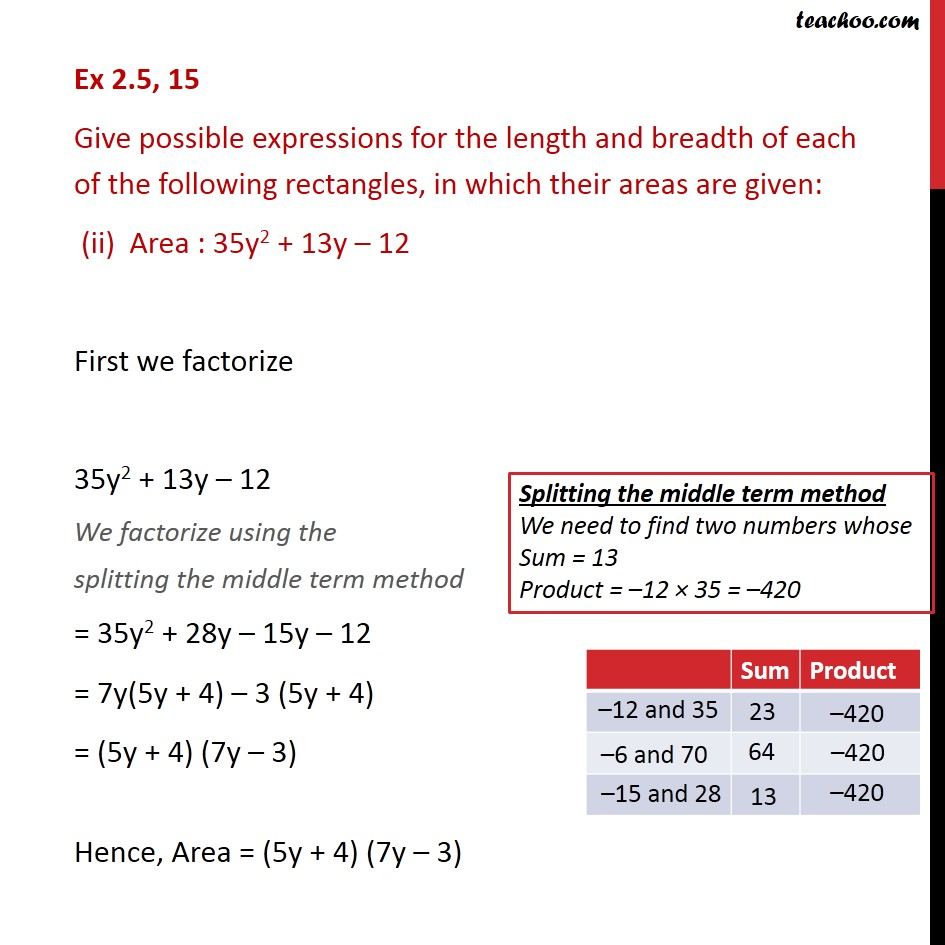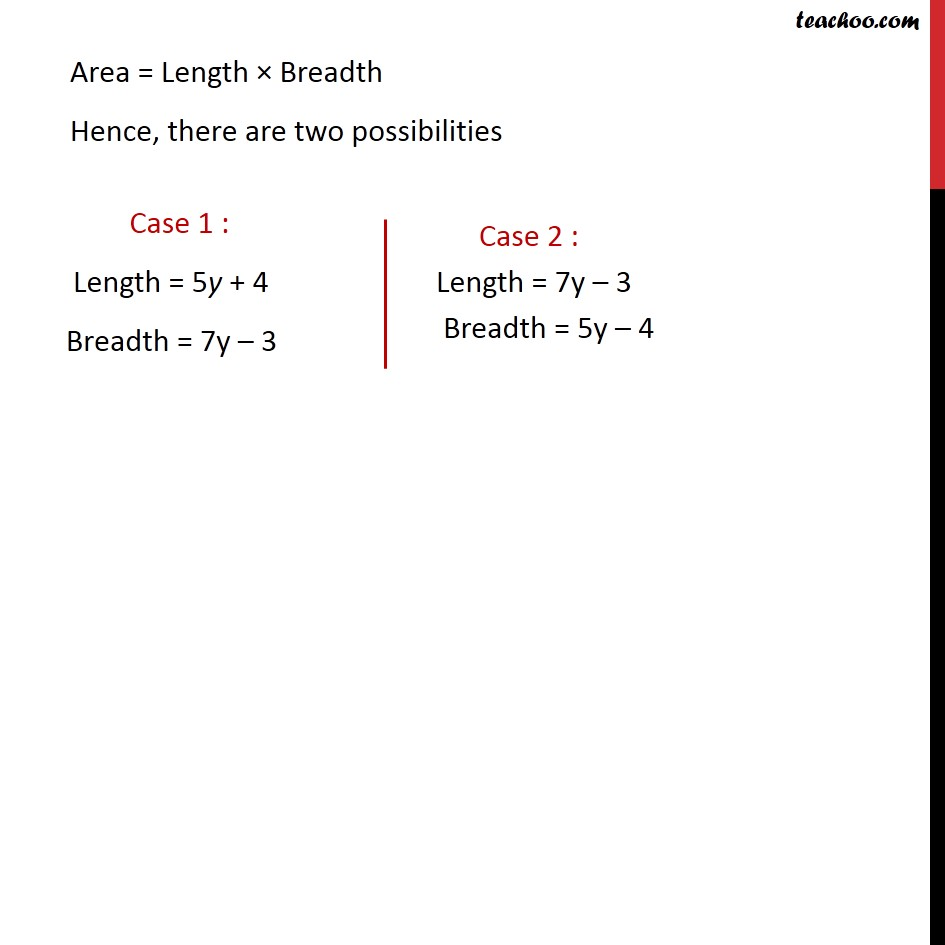Ex 2.5

Chapter 2 Class 9 Polynomials (Term 2)
Serial order wise### Transcript

Ex 2.5, 15 Give possible expressions for the length and breadth of each of the following rectangles, in which their areas are given: (ii) Area : 35y2 + 13y – 12 First we factorize 35y2 + 13y – 12 We factorize using the splitting the middle term method = 35y2 + 28y – 15y – 12 = 7y(5y + 4) – 3 (5y + 4) = (5y + 4) (7y – 3) Hence, Area = (5y + 4) (7y – 3) Area = Length × Breadth Hence, there are two possibilities